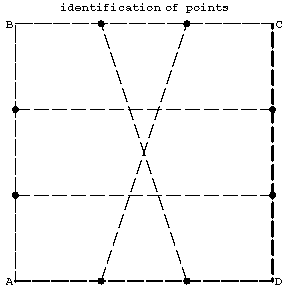# Klein surface

(diff) ← Older revision | Latest revision (diff) | Newer revision → (diff)

Klein bottle

A closed one-sided surface of genus 1 (see Fig. a, a, b).Figure: k055510a

The Klein surface can be obtained from a square $ABCD$ (see Fig. b) by identifying the points of the line segments $AB$ and $CD$ lying on the lines parallel to the side $AD$ and the points of the segments $BC$ and $AD$ symmetric with respect to the centre of the square $ABCD$.Figure: k055510b

The Klein surface can be topologically imbedded in the $4$-dimensional Euclidean space, but not in $3$-dimensional space.

Attention was drawn to the Klein surface by F. Klein (1874).

A Klein bottle can be obtained by glueing together two crosscaps (Möbius bands, cf. Möbius strip) along their boundaries. The homology of the Klein bottle $K$ is: $H_0(K;\mathbf Z)=\mathbf Z$, $H_1(K;\mathbf Z)=\mathbf Z\oplus\mathbf Z/(2)$, $H_2(K;\mathbf Z)=0$. Its Euler characteristic is $\chi(K)=0$. Together with the torus it is the only smooth $2$-dimensional surface which admits deformations of the identity mapping that have no fixed points.